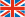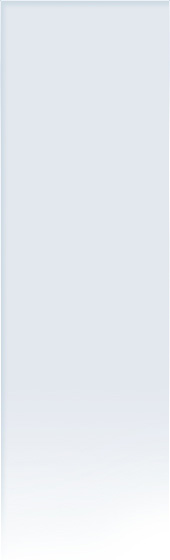# Typesetting formulas with RagTime

03/20/2018

Typesetting formulas has long been a current topic for RagTime. Since RagTime does not have its own formula editor, we looked at the possibilities.
This article only covers the Mac. If Windows users have additions to this, we would be grateful for feedback.

There are various formula editors in various price classes and functions. Besides the free “Daum Equation Editor”, which only allows exporting formulas as PNG, and the well-known MathType for \$97 (as of March 2018), we have now looked at the Equation Maker, which is discussed in the following text. The software is available in the Apple App Store for \$7.99.

Equation Maker

The main window shows the formula and the associated LaTeX code in which the formula is entered: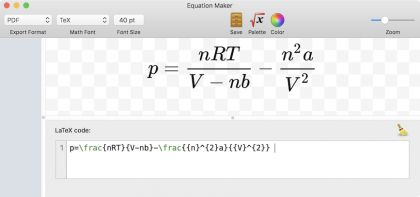Even beginners get along well with it, because important commands or formula components are generated via a palette: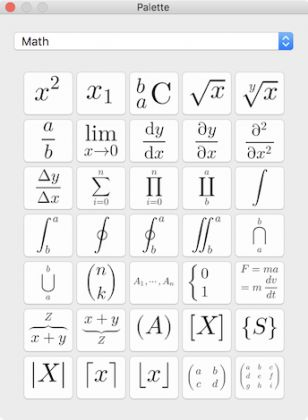So a click on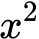inserts the command {x}^{2}. Next, you can replace x or 2 with the desired characters.
If you now select x and click, you get {left(    A   right)}^{2}, which is displayed as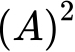. Now you can replace A e.g. by a fraction (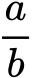), which leads to. Note that the parentheses are set to the same height, which is the case when left( and right) are used. If simply typed ( and ), the output would look like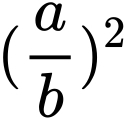.
In this way, you can also set complex formulas, which can then be saved as PDF files in the Finder using “Drag & Drop”, for example.

In RagTime the data can also be transferred, whereby the import format PDF should be selected: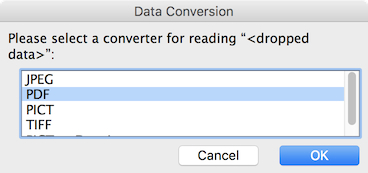If the formulas are saved as PDF in the Finder, you can drag them back into Equation Maker to change the formula later.

Here are some sample formulas (source: Wikipedia):

Coefficient of thermal expansionEnergy balance for any open systemElectrical mobility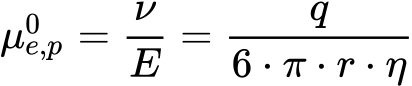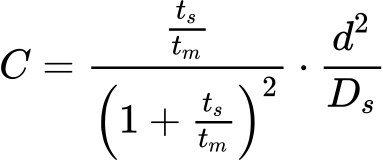or also chemical reactions, such as:

Autoprotolysis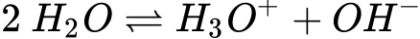Prussian blue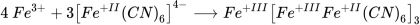Acetaldehyde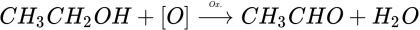RagTime.de Development GmbH

Steinriede 3
30827 Garbsen
Germany

Tel.: +49 5131 70 93 6-0

Simply productive

RagTime 6

Inventory and Foyer

Individual content, formats, fonts, and colors are listed well arranged in the Inventory. Frequently used documents and form can be accessed easily from within the Foyer.